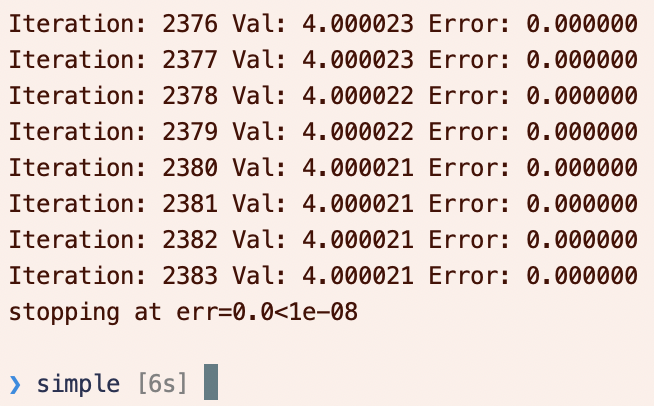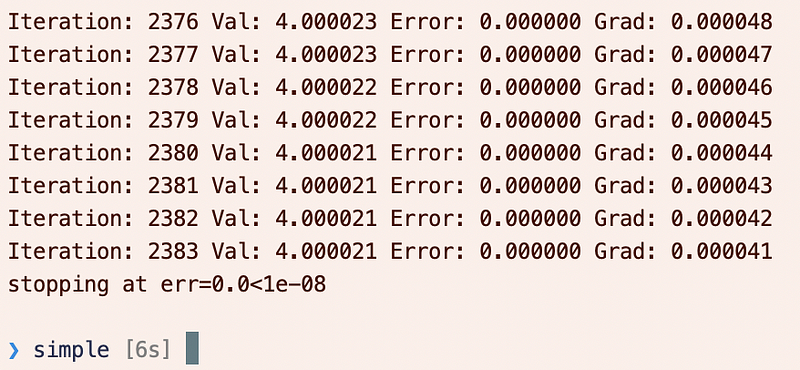A simple optimization example with Tensorflow 2.0.

A simple optimization example with Tensorflow 2.0.I’ve often used `tf.GradientTape` from StackOverflow snippets, hastily glued together. I’ve also often used `tf.GradientTape` , and the `minimize` method usually appears. Finally I’ve separated the two in my mind in a simple application: optimizing a 1D function, e.g. `(x-4)**2` in one scalar variable `x`.

The relationship between the two approaches is actually quite simple:

• One optimization method is to use the `minimize` method, as documented here, which performs two steps:
1. It uses `tf.GradientTape` to calculate the gradient.
2. It uses `apply_gradients` to apply the gradients to the parameters.
• The other method is to unpack the action of the `minimize` method and manually perform these two steps.

### Optimization using `minimize`

As a simple example, consider minimizing `(x-4)**2` in one scalar variable `x` . To do so, we define a `loss_function()` , which must take no arguments.

• `loss_function` is the loss function
• `run` is the run function starting from an initial guess.
• Iteratively, we apply the `opt.minimize(loss_function, var_list=[x])` function to minimize the loss function with respect to the variable list.
• We stop if the error tolerance is sufficiently low.
• `minimize` automatically updates the iterations (actually: `apply_gradients` is updating the iterations, which is called by `minimize` ), and we stop if we hit the limit.

The output should be something like the following:Alternatively, we can use the `tf.GradientTape` and `apply_gradients` methods explicitly in place of the `minimize` method.

• We no longer need a loss function.
• We use `with tf.GradientTape(persistent=False) as t` to create the tape, and then `t.gradient(y,[x])` to calculate the gradient in `y` with respect to `x` .
• Note: you may be surprised that we can exit the `with` indentation and still access the tape `t` ! In this case, you need to familiarize yourself with “blocks” as discussed here. The scope of variables extends throughout a block, which is either a module, function or a class. Since the `with` statement is not one of these, then you can still access the gradient tape! You may have previously seen the `with` statement used like this:
``````with open(fname,"r") as f:
...
``````

But even here, even though the file is closed when the `with` statement ends, the variable `f` is not out of scope.

• We apply the gradients to `x` with `opt.apply_gradients(zip(gradients,[x]))` .

The output should be similar, but now we also have gradient information:### Final thoughts

Pretty simple, really!

A couple last notes on `tf.GradientTape` :

• Most times you do not need to call `t.watch(x)` — from the documentation, “By default GradientTape will automatically watch any trainable variables that are accessed inside the context.” You can disable this in the constructor of the `tf.GradientTape` .
• When do you need to use `persistent=True` : you really only need this when you have defined an intermediate variable that you also want the gradient with respect to. For example, the following will **not** work:
``````with tf.GradientTape(persistent=False) as t:
z = x-4
y = z**2
``````

Even though `t` is still in scope after the `with` block (see discussion above), from the documentation: “By default, the resources held by a GradientTape are released as soon as GradientTape.gradient() method is called.”. So the second `gradient` call will fail.

``````with tf.GradientTape(persistent=True) as t:
z = x-4
y = z**2
``````# Don't forget now to do garbage collection when done computing gradients!
Don’t forget the `del t` after you’ve computed all the gradients you’re interested in.